Next: 3-d problems Up: The diffusion equation Previous: An example 2-d diffusion

## An example 2-d solution of the diffusion equation

Let us now solve the diffusion equation in 2-d using the finite difference technique discussed above. We seek the solution of Eq. (214) in the region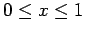and, subject to the following initial condition at: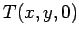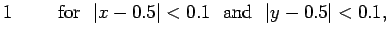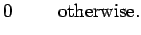(229)

The boundary conditions are simply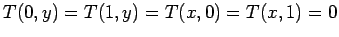.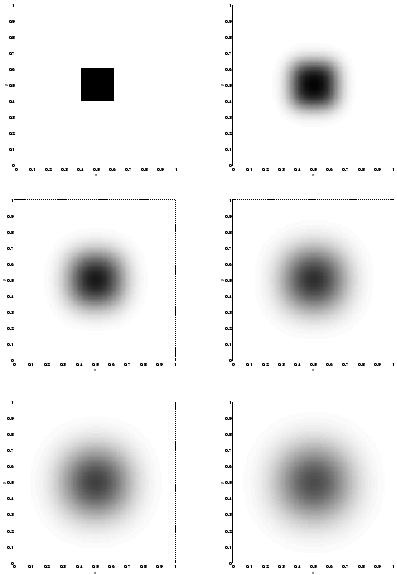Figure 74 shows the evolution of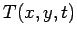for a calculation performed with the previously listed 2-d diffusion equation solver using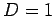,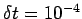, and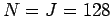.Next: 3-d problems Up: The diffusion equation Previous: An example 2-d diffusion
Richard Fitzpatrick 2006-03-29